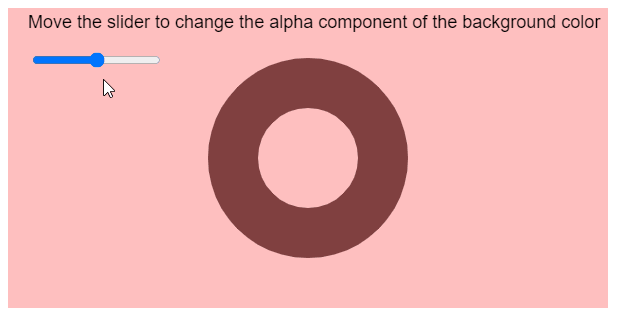Related Articles
p5.Color setAlpha() Method
• Last Updated : 28 Jul, 2020

The setAlpha() method in p5.js is used to set the alpha component of a p5.Color object. The range of the alpha value is dependent on the current color mode. In the default RGB color mode, the value can be between 0 and 255.

Syntax:

```setAlpha( alpha )
```

Parameters: This method accepts a single parameter as mentioned above and described below:

• alpha: It is a number that denotes the new alpha value.

The example below illustrates the setAlpha() method in p5.js:

Example 1:

## Javascript

 `function` `setup() {``  ``createCanvas(600, 300);`` ` `  ``alphaSlider = createSlider(0, 255, 128);``  ``alphaSlider.position(30, 50);``}`` ` `function` `draw() {``  ``clear();``  ``textSize(18);`` ` `  ``// Get the alpha value from the slider``  ``let alphaValue = alphaSlider.value();`` ` `  ``let newColor = color(128, 255, 128);`` ` `  ``// Set the alpha value of the color``  ``newColor.setAlpha(alphaValue);``   ` `  ``background(newColor);``  ``fill(``'black'``);``  ``text(``"Move the slider to change the "` `+``       ``"alpha component of the background color"``,``       ``20, 20);``   ` `}`

Output:Example 2:

## Javascript

 `function` `setup() {``  ``createCanvas(600, 300);`` ` `  ``alphaSlider = createSlider(0, 255, 128);``  ``alphaSlider.position(30, 50);``}`` ` `function` `draw() {``  ``clear();``  ``textSize(18);`` ` `  ``noFill();``  ``stroke(0);``  ``strokeWeight(50);``  ``ellipse(width / 2, height / 2, 150);``  ``strokeWeight(1);`` ` `  ``// Get the alpha value from the slider``  ``let alphaValue = alphaSlider.value();`` ` `  ``let newColor = color(255, 128, 128);`` ` `  ``// Set the alpha value of the color``  ``newColor.setAlpha(alphaValue);``   ` `  ``noStroke();``  ``background(newColor);``  ``fill(``'black'``);``  ``text(``    ``"Move the slider to change the "` `+``    ``"alpha component of the background color"``,``    ``20, 20);``   ` `}`

Output:Online editor: https://editor.p5js.org/

My Personal Notes arrow_drop_up